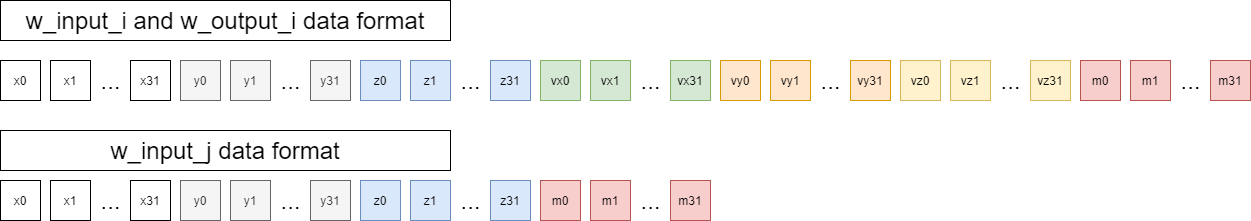# A Single Nbody() Kernel - 2023.1 English

## Vitis Tutorials: AI Engine Development

Document ID
XD100
Release Date
2023-09-20
Version
2023.1 English

Review the `src/nbody.cc` file. It contains the implementation of a single AI Engine kernel mapped to a single AI Engine tile called `nbody()`. This kernel takes in the `x y z vx vy vz m` values for 32 particles, computes the N-Body gravity equations for a single timestep, and outputs the new `x y z vx vy vz m` values for the 32 particles. This kernel takes in two inputs: `w_input_i` and `w_input_j`. The `w_input_i` window contains the `x y z vx vy vz m` floating point values for 32 particles. The `w_input_j` window contains the only `x y z m` floating-point values for the same 32 particles. This kernel produces one output: `w_output_i` which contains the new `x y z vx vy vz m` floating-point values for the 32 particles in the next timestep.

name number of 32-bit data values
`w_input_i` 32 * 7 = 224
`w_input_j` 32 * 4 = 128
`w_output_i` 32 * 7 = 224The `nbody()` kernel is sectioned into two major `for` loops. The first major `for` loop (around lines 38-61) calculates the new `x y z` positions for the 32 particles. The second major `for` loop (around lines 64-202) calculates the new `vx vy vz` velocities for the 32 particles. The output mass (`m`) values remain the same as the inputs. The `w_output_i` window is then sent to the `transmit_new_i` kernel (source: `src/transmit_new_i.cc`) to be written to the final output window.